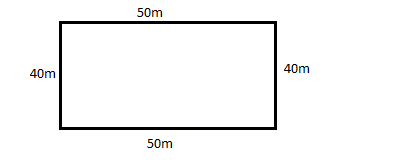Courses
Courses for Kids
Free study material
Free LIVE classes
MoreLIVE
Join Vedantu’s FREE Mastercalss

# A rectangular field is 50m $\times$ 40m. Ramesh goes 20 times around it. How much distance does he cover?Verified
361.5k+ views
Hint: Perimeter of any shape is equal to the length around the shape.

Given that a field is in rectangular shape with dimensions 50m $\times$ 40m
$\Rightarrow$ length = 50m and breadth 40m.
The rectangular field is similar to the below figure.We know that the perimeter of any rectangle is equal to the sum of measurements of all sides.
$\Rightarrow$ Perimeter of the given rectangular field = 40 + 50 + 40 + 50 = 180m
The distance covered by a person when he runs around that field = perimeter of field = 180m
Ramesh goes around the field 20 times. That means he covered the perimeter 20 times.
$\therefore$ Distance covered by Ramesh = $20 \times 180 = 3600m$

Note: When a surface is in rectangular shape with length ‘l’ and breadth ‘b’, then perimeter = 2(l + b). We can directly use this formula to calculate the perimeter of the rectangle.
Last updated date: 22nd Sep 2023
Total views: 361.5k
Views today: 9.61k